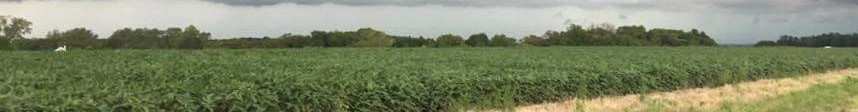# Equations and Information

Master Book Found In Main Office.

# Weight and Measurers Conversions

## Weight

16 ounces = 1 pound = 453.6 grams
1 gallon water = 8.34 pounds = 3.78 liters

## Liquid Measure

1 fluid ounce = 2 tablespoons = 29.57 milliliters
16 fluid ounces = 1 pint = 2 cups
8 pints = 4 quarts = 1 gallon

## Length

3 feet = 1 yard = 91.44 centimeters
16.5 feet = 1 rod
5,280 feet = 1 mile = 1.61 kilometers
320 rods = 1 mile

## Area

9 square feet = 1 square yard
43,560 square feet = 1 acre = 160 square rods
1 acre = 0.405 hectare
640 acres = 1 square mile

## Speed

88 feet per minute = 1 mph
1 mph = 1.61 kilometer per hour

## Volume

27 cubic feet = 1 cubic yard
1 cubic foot = 1,728 cubic inches = 7.48 gallons
1 gallon = 231 cubic inches
1 cubic foot = 0.028 cubic meters

## Common Abbreviations and Terms

FPM = Feet Per Minute
GPA = Gallons Per Acre
GPH = Gallons Per Hour
GPM = Gallons Per Minute
mph = Miles Per Hour
psi = Pounds Per Square Inch
RPM = Revolutions Per Minute

# Sprayer Equations

## Flow Rate

GPM = (GPA X MPH X W) / 5940

## Horsepower Needed

Hp = (GPM X PSI) / (1714 X Eff)

## Tank-Field Capacity

Acres per Tank = (tank volume) / (GPA)